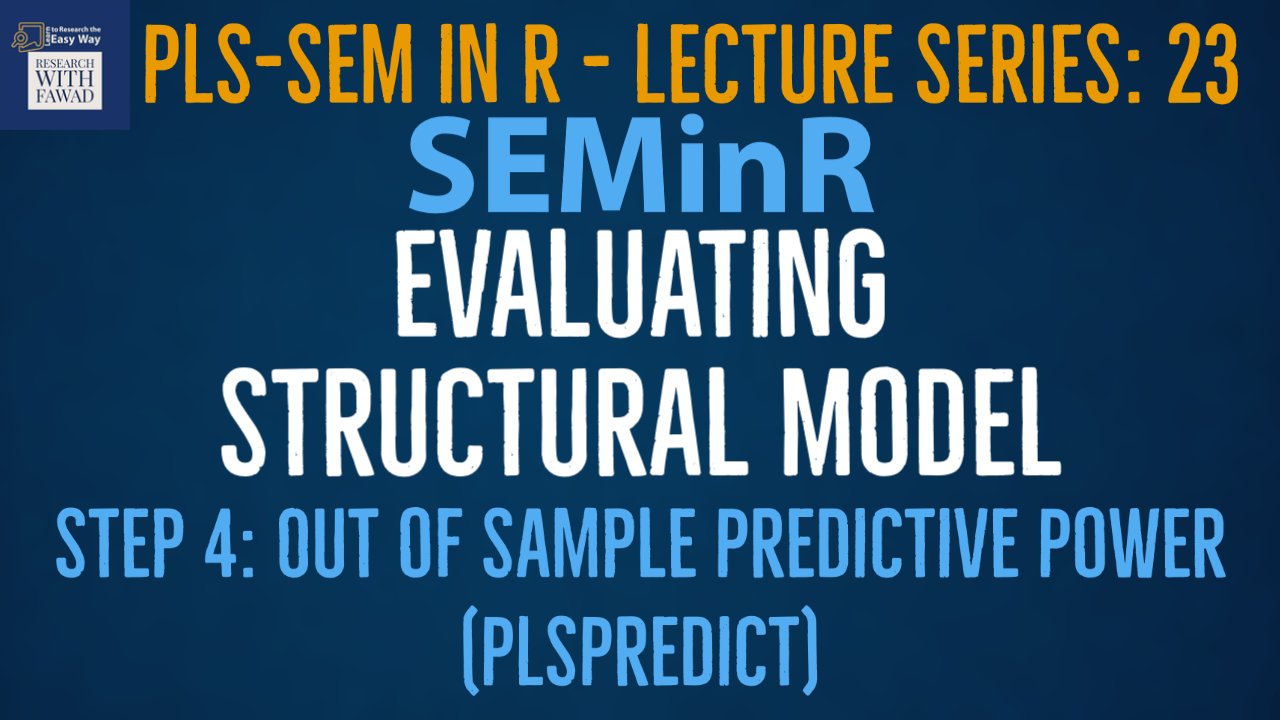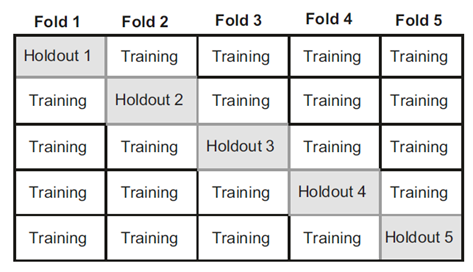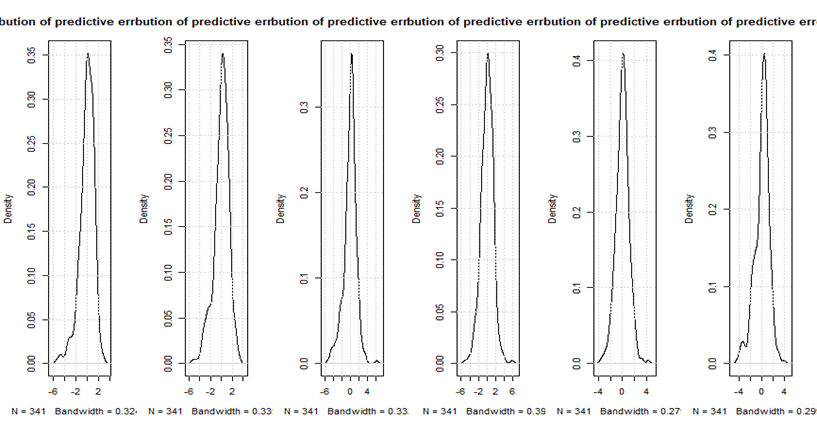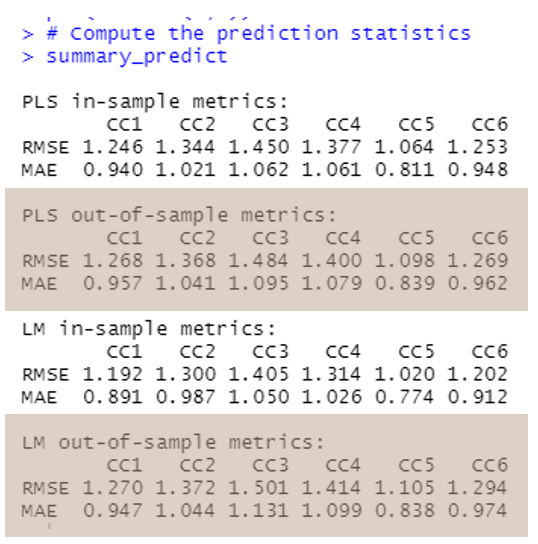# SEMinR Lecture Series - Out of Sample Predictive Power - PLSPredict### SEMinR Lecture Series

This session is focused on Step 4 of the structural model evaluation that is Out of Sample Predictive Power, assessed through PLSPredict.

## Evaluating Structural Model

• Once you have confirmed that the measurement of constructs is reliable and valid, the next step addresses the assessment of the structural model results.
• Figure shows a systematic approach to the structural model assessment.
• This is step 4 examining the model’s predictive power.

## Step 4: Assess the Model’s Predictive Power

• Many researchers interpret the R-Sq statistic as a measure of their model’s predictive power (Sarstedt & Danks, 2021; Shmueli & Koppius, 2011). This interpretation is not entirely correct, however, since the R-Sq only indicates the model’s in-sample explanatory power.
• “In sample” refers to the data that you have, and “out of sample” to the data you don’t have but want to forecast or estimate.
• It says nothing about the model’s predictive power (Chin et al., 2020; Hair & Sarstedt, 2021), also referred to as out-of-sample predictive power, which indicates a model’s ability to predict new or future observations. Addressing this concern, Shmueli, Ray, Estrada, and Chatla (2016) introduced PLSpredict, a procedure for out-of-sample prediction.
• Execution of PLSpredict involves estimating the model on a training sample and evaluating its predictive performance on a holdout sample (Shmueli et al., 2019).
• Note that the holdout sample is separated from the total sample before executing the initial analysis on the training sample data, so it includes data that were not used in the model estimation.
• Researchers need to make sure that the training sample for each fold meets minimum sample size guidelines (e.g., by following the inverse square root method).
• Execution of PLSpredict involves estimating the model on a training sample and evaluating its predictive performance on a holdout sample (Shmueli et al., 2019). Note that the holdout sample is separated from the total sample before executing the initial analysis on the training sample data.
• PLSpredict executes k-fold cross-validation. A fold is a subgroup of the total sample, while k is the number of subgroups. That is, the total dataset is randomly split into k equally sized subsets of data.
• For example, a cross-validation based on k = 5 folds splits the sample into five equally sized data subsets (i.e., groups of data). PLSpredict then combines k-1 subsets (i.e., four groups of data) into a single training sample that is used to predict the remaining fifth subset.
• Holdout Sample is Predictied based on the training sample.
• Each case in every holdout sample has a predicted value estimated with the respective training sample.
• A training sample is a portion of the overall dataset used to estimate the model parameters (e.g. the path coefficients, indicator weights, and loadings). The remaining part of the dataset not used for model estimation is referred to as the holdout sample.
• The training dataset is used to ESTIMATE (i.e. train) the weights and paths of our model, and we use these estimated weights to predict the outcomes of the holdout sample.
• We then evaluate the prediction metrics (MSE, MAE, etc.) of the predictions on the holdout sample We do not compare predictions of training and holdout samples.## Cross validation Process

• Figure visualizes the cross-validation process. Shmueli et al. (2019) recommend setting k = 10
• For each validation, each subset is used as a holdout sample. PLSpredict then combines k-1 subsets (i.e. 4) into a single training sample to predict the remaining subset, which represents the holdout sample for the first cross-validation run.
• The cross-validation process is then repeated k times (the folds), with each of the k subsets used exactly once as the holdout sample. Figure illustrates this concept for k=5. In Fold 1, the first subset (Holdout 1) is excluded from the analysis and the model is estimated using the training data, which is made up by combining Folds 2 through 5 into a single sample.
• The estimates are then used to predict the holdout sample (Holdout 1 predicted).

This process is repeated for Fold 2 through Fold 5, yielding predictions for all five holdout samples. For example, Fold 2 becomes the holdout sample and the training sample consists of Fold 1 and Folds 3, 4 and 5.

• Consequently, each case in every holdout sample has a predicted value based on a model in which that case was not used to estimate the model parameters. The accuracy of these predictions is then summarized in the prediction statistics.

• The generation of the k subsets of data is a random process and can therefore result in extreme partitions that potentially lead to abnormal solutions. To avoid such abnormal solutions, researchers should run PLSpredict multiple times.
• To assess a model’s predictive power, researchers can draw on several prediction statistics that quantify the amount of prediction error in the indicators of a particular endogenous construct.
• Error is not an error (as in a mistake). It is a residual, the lower the better, this is the difference between actual values and the predicted values.
• The most popular metric to quantify the degree of prediction error is the root-mean-square error (RMSE).
• Another popular metric is the mean absolute error (MAE).

## RMSE or MAE

• To assess a model’s predictive power, researchers can draw on several prediction statistics that quantify the amount of prediction error in the indicators of a particular endogenous construct.
• Error is not an error (as in a mistake). It is a residual, the lower the better, this is the difference between actual values and the predicted values.
• The most popular metric to quantify the degree of prediction error is the root-mean-square error (RMSE).
• Another popular metric is the mean absolute error (MAE).
• In most instances, researchers should use the RMSE to examine a model’s predictive power. But if the prediction error distribution is highly nonsymmetric, as evidenced in a long left or right tail in the distribution of prediction errors (Danks & Ray, 2018), the MAE is the more appropriate prediction statistic (Shmueli et al., 2019).
• To interpret these metrics, researchers need to compare each indicator’s RMSE (or MAE) values with a naïve linear regression model (LM) benchmark.
• The LM benchmark values are obtained by running a linear regression of each of the dependent construct’s indicators on the indicators of the exogenous constructs in the PLS path model (Danks & Ray, 2018). In comparing the RMSE (or MAE) values with the LM values, the following guidelines apply (Shmueli et al., 2019):
• If all indicators in the PLS-SEM analysis have lower RMSE (or MAE) value compared to the naïve LM benchmark, the model has high predictive power.

• If the majority (or the same number) of indicators in the PLS-SEM analysis yields smaller prediction errors compared to the LM, this indicates a medium predictive power.

• If a minority of the dependent construct’s indicators produce lower PLS-SEM prediction errors compared to the naïve LM benchmark, this indicates the model has low predictive power.

• If the PLS-SEM analysis (compared to the LM) yields lower prediction errors in terms of the RMSE (or the MAE) for none of the indicators, this indicates the model lacks predictive power.

## How to Generate Predictions?

• An important decision when using PLSpredict is how to generate the predictions when the PLS path model includes a mediator construct (mediation is discussed further in future videos), which is both a predictor to the outcome and itself the outcome of an antecedent.
• SEMinR offers two alternatives to generate predictions in such a model setup (Shmueli et al., 2016). Researchers can choose to generate predictions using either the direct antecedents (DAs) or the earliest antecedents (EAs).
• In the DA approach, PLSpredict would consider both the antecedent and the mediator as predictors of outcome constructs, whereas in the EA approach, the mediator would be excluded from the analysis.
• Danks (2021) presents simulation evidence that the DA approach generates predictions with the highest accuracy. Hence, DA is recommended.

## Illustration

• To do so, we first have to generate the predictions using the predict_pls() function. .
• We run the PLSpredict procedure with k = 10 folds and ten repetitions and thus set noFolds = 10, and reps = 10. In addition, we use the predict_DA approach.
• Finally, we summarize the PLSpredict model and assign the output to the summary_predict object:
``````# Generate the model predictions based on PLS Estimate
predict_model <- predict_pls(
model = simple_model,
technique = predict_DA,
noFolds = 10,
reps = 10)
# Summarize the prediction results
summary_predict <- summary(predict_model)``````

### Distribution of Prediction Errors

• The distributions of the prediction errors need to be assessed to decide the best metric for evaluating predictive power.
• If the prediction error is highly skewed, the MAE is a more appropriate metric than the RMSE. In order to assess the distribution of predictive error, we use the plot() function on the object summary_predict and specify the indicator argument to the indicators of interest.
• We should focus on the key outcome construct Collaborative Culture (as it is the ultimate dependent variable) and evaluate the indicators CC1-CC6.
• First, we set the number of plots to display in the output to three plots arranged horizontally using the par(mfrow=c(1,3)) command. Remember to set par(mfrow=c(1,1))after outputting the plots; otherwise, all future plots will be arranged horizontally in a sequence of three:
``````# Analyze the distribution of prediction error
par(mfrow=c(1,6))
plot(summary_predict,
indicator = "CC1")
plot(summary_predict,
indicator = "CC2")
plot(summary_predict,
indicator = "CC3")
plot(summary_predict,
indicator = "CC4")
plot(summary_predict,
indicator = "CC5")
plot(summary_predict,
indicator = "CC6")
par(mfrow=c(1,1))
``````

### Prediction Error Distribution

• The results in . Fig. show that while all plots have a tail and are slightly skewed, the prediction error distributions are rather symmetric.
• We should therefore use the RMSE for our assessment of prediction errors.### Output

• We can investigate the RMSE and MAE values by calling the summary_predict object.
``````# Compute the prediction statistics
summary_predict``````

## Results

• Analyzing the construct’s indicators (Fig.),

• we find that the PLS path model has lower out-of-sample predictive error (RMSE) compared to the naïve LM model benchmark for all indicators (sections: PLS out-of-sample metrics and LM out-of-sample metrics)

• Accordingly, we conclude that the model has a high predictive power.